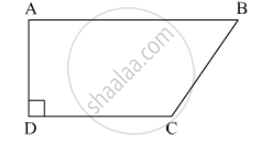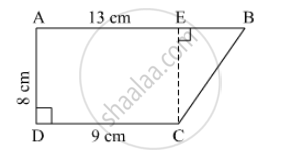Advertisement Remove all ads

# In ☐ Abcd, L(Ab) = 13 Cm, L(Dc) = 9 Cm, L(Ad) = 8 Cm, Find the Area of ☐ Abcd. - Mathematics

Sum

In ☐ ABCD, l(AB) = 13 cm, l(DC) = 9 cm, l(AD) = 8 cm, find the area of ☐ ABCD.Advertisement Remove all ads

#### SolutionDraw perpendicular from c to line AB. Name the point E.
CE = AD = 8 cm

EB = AB - AE = AB - CD = 13 - 9 = 4cm

Area of rectangle AECD = l x b = 9 x 8 = 72 cm²

Area of Triangle BEC = 1/2 x b x h = 1/2 x 8 x 4 = 16 cm²

Area of ☐ ABCD = Area of AECD + Area of triangle BEC = 72 + 16 = 88 cm2

Is there an error in this question or solution?
Advertisement Remove all ads

#### APPEARS IN

Balbharati Mathematics 8th Standard Maharashtra State Board
Chapter 15 Area
Practice Set 15.3 | Q 1 | Page 99
Advertisement Remove all ads
Advertisement Remove all ads
Share
Notifications

View all notifications

Forgot password?